## General Question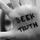Asked by seVen (3472) November 10th, 2008
Observing members: 0Composing members: 0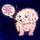Trying to break it down and find a part of the number I can calculate easily, then adding that to the rest of it. (Did that make sense?) Like if I want to multiply 24×8 I’ll do 8×20 + 8×4.

Allie (17441)“Great Answer” (4) Flag as…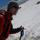Here are 3 cool tips I just found:

1. Round Off to a Quick Approximation

First determine a level of accuracy you are happy with, then round the numbers appropriately before making the calculation. This is also useful to determine a quick ballpark figure before doing the actual calculation.

2. Break Up Into Parts

Say you have to multiply 613 by three. Try multiplying first 600 by 3, then 10 by 3 then 3 by 3 and adding the results.

4. Quickly Find Three Common Percentages

20% -> Divide the number by 5
33.3% -> Divide the number by 3
50% -> Divide the number by 2

Source

TheBox193 (992)“Great Answer” (3) Flag as…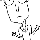If I want to be correct, I use a calculator.

If I only want to be in the right ballpark, I’ll round all the numbers to the nearest power of 10 and then do the math. In Allie’s example, I’d round 24×8 to 10×10 and say the answer is “over a hundred.” This is TheBox193’s method #1 with a level of accuracy of “not very.”

If I want someone else to figure it out, I’ll declare some arbitrary number to be the answer until someone gets annoyed enough to prove me wrong.

jasongarrett (1736)“Great Answer” (2) Flag as…jason: Wouldn’t it be more accurate to call 8 a 10 and 24 a 20? Then do 20×10 and say it’s around 200? Cause using 10×10 leaves you nearly 100 off.

…but I get what you’re saying.

Allie (17441)“Great Answer” (0) Flag as…Yeah, the order-of-magnitude thing works better with bigger numbers.

jasongarrett (1736)“Great Answer” (0) Flag as…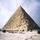I agree, break the number up, do the tens first, then bust up the rest of it. This will give you the exact answer quickly without ballparking it.

psyla (2544)“Great Answer” (0) Flag as…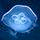24 times 12

4times 2 gives 8 (colum multiply)
cross multiply both numbers and add them 2times 2 + 4times 1 = 8
finally 2times 1 gives 2 (colum multiply)

thus 288

from speed math

mea05key (1812)“Great Answer” (0) Flag as…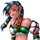Base10 is my friend.

12+4 = 10 + 6 to me.
2347×7 = 2000×7 + 347

Like everyone here, I just break it down into simple chunks and then mush them back together.

squirbel (4292)“Great Answer” (1) Flag as…@squirbel, wouldn’t that be
2000×7 plus
300×7 plus
40×7 plus
7×7.
Just checking LOL

stevenb (3816)“Great Answer” (0) Flag as…I am all about the 10s.

9×35 gets converted to
10×35 = 350 then
350 – 35 = 315

augustlan (47701)“Great Answer” (1) Flag as…or